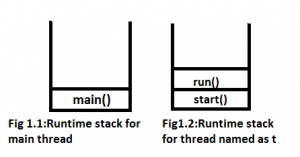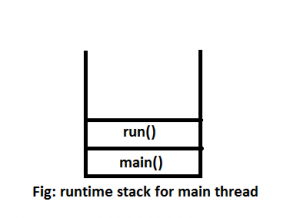Open In App

Overloading of run() method is possible. But Thread class start() method can invoke no-argument method. The other overloaded method we have to call explicitly like a normal method call.

 `// Java Program to illustrate the behavior of``// run() method overloading``class` `Geeks ``extends` `Thread {``    ``public` `void` `run()``    ``{``        ``System.out.println(``"GeeksforGeeks"``);``    ``}``    ``public` `void` `run(``int` `i)``    ``{``        ``System.out.println(``"Bishal"``);``    ``}``} `` ` `class` `Test {``    ``public` `static` `void` `main(String[] args)``    ``{``        ``Geeks t = ``new` `Geeks();``        ``t.start();``    ``}``}`

Output:

`GeeksforGeeks`

Runtime stack provided by JVM for the above program:NOTE : Overloaded run() method will be ignored by the Thread class unless we call it ourselves. The Thread class expect a run() with no-arg and that will execute in a separate call stack after the thread has been started. With run(int i), it will not start any separate call stack even if we call it directly. It will be in the same call stack like any other method (if you call from run() method).

Example:

 `// Java Program to illustrate the execution of``// program using main thread``class` `Geeks ``extends` `Thread {``    ``public` `void` `run()``    ``{``        ``System.out.println(``"GeeksforGeeks"``);``    ``}``    ``public` `void` `run(``int` `i)``    ``{``        ``System.out.println(``"Bishal"``);``    ``}``} `` ` `class` `Test ``extends` `Geeks {``    ``public` `static` `void` `main(String[] args)``    ``{``        ``Geeks t = ``new` `Geeks();``        ``t.run(``1``);``    ``}``}`

Output:

```Bishal
```
Runtime stack provided by JVM for the above program: